# JP4685509B2 - AC motor drive control device and drive control method - Google Patents

## Info

Publication number
JP4685509B2
JP4685509B2 JP2005151165A JP2005151165A JP4685509B2 JP 4685509 B2 JP4685509 B2 JP 4685509B2 JP 2005151165 A JP2005151165 A JP 2005151165A JP 2005151165 A JP2005151165 A JP 2005151165A JP 4685509 B2 JP4685509 B2 JP 4685509B2
Authority
JP
Japan
Prior art keywords
value
estimated
torque value
voltage
calculating
Prior art date
Legal status (The legal status is an assumption and is not a legal conclusion. Google has not performed a legal analysis and makes no representation as to the accuracy of the status listed.)
Active
Application number
JP2005151165A
Other languages
Japanese (ja)
Other versions
JP2006054995A (en
Inventor

Original Assignee
トヨタ自動車株式会社

Priority date (The priority date is an assumption and is not a legal conclusion. Google has not performed a legal analysis and makes no representation as to the accuracy of the date listed.)
Filing date
Publication date
Priority to JP2004204792 priority Critical
Application filed by トヨタ自動車株式会社, 株式会社豊田中央研究所 filed Critical トヨタ自動車株式会社
Priority to JP2005151165A priority patent/JP4685509B2/en
Publication of JP2006054995A publication Critical patent/JP2006054995A/en
Application granted granted Critical
Publication of JP4685509B2 publication Critical patent/JP4685509B2/en
Active legal-status Critical Current
Anticipated expiration legal-status Critical

• 238000004364 calculation methods Methods 0.000 claims description 58
• 238000000034 methods Methods 0.000 claims description 9
• 230000001276 controlling effects Effects 0.000 claims description 5
• 230000004044 response Effects 0.000 description 12
• 238000010586 diagrams Methods 0.000 description 7
• 230000004043 responsiveness Effects 0.000 description 7
• 238000006243 chemical reactions Methods 0.000 description 5
• 230000000875 corresponding Effects 0.000 description 5
• 230000004907 flux Effects 0.000 description 4
• 230000001052 transient Effects 0.000 description 2
• 238000004804 winding Methods 0.000 description 2
• 241001442055 Vipera berus Species 0.000 description 1
• 230000000694 effects Effects 0.000 description 1
• 230000005284 excitation Effects 0.000 description 1
• 239000000203 mixtures Substances 0.000 description 1
• 230000000630 rising Effects 0.000 description 1
• 239000007787 solids Substances 0.000 description 1

## Images

•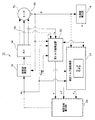•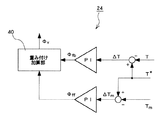•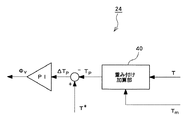•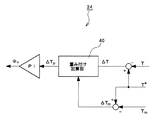••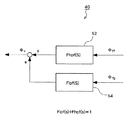•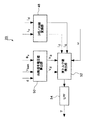•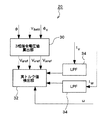•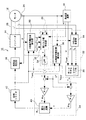•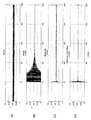•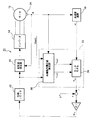•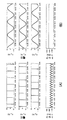•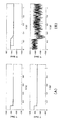## Classifications

• HELECTRICITY
• H02GENERATION; CONVERSION OR DISTRIBUTION OF ELECTRIC POWER
• H02PCONTROL OR REGULATION OF ELECTRIC MOTORS, ELECTRIC GENERATORS OR DYNAMO-ELECTRIC CONVERTERS; CONTROLLING TRANSFORMERS, REACTORS OR CHOKE COILS
• H02P21/00Arrangements or methods for the control of electric machines by vector control, e.g. by control of field orientation
• H02P21/22Current control, e.g. using a current control loop

## Description

The present invention relates to a drive control device and a drive control method for controlling an AC motor that is rotationally driven by application of a rectangular wave voltage.

In order to output a given command torque from an electric motor, a technique for controlling an applied voltage to the electric motor is widely known. For example, Patent Document 1 discloses a drive control device that feeds back an output torque value of an electric motor and calculates a voltage phase that eliminates a deviation between the output torque value and a command torque value. In Patent Document 1, an output torque value is acquired based on each phase current value detected by a detector, a command voltage value, and an angular velocity. In Patent Document 2, an output torque value is acquired by a torque sensor.

Patent Document 3 below discloses an electric motor control device that controls an inverter phase angle so that a command torque value can be output. This obtains the torque correction angle based on the deviation between the output torque value and the command torque value, and the angle (voltage vector angle) of the voltage vector with respect to the magnetic flux axis direction using the motor model simulating the motor and the command torque value. To do. Then, the inverter phase is calculated based on the acquired torque correction angle, voltage vector angle, and the like.

JP 2001-28892 A JP 2000-50689 A Japanese Patent Laid-Open No. 10-14273

By the way, normally, a low-pass filter for removing noise is provided in the output torque detection process. In particular, when the output torque value is calculated based on the detected value of each phase current value, since a harmonic component is included in the detected current value, noise removal by a low-pass filter is essential. However, when a low-pass filter is used, a time delay occurs in the obtained output torque value, and torque response is reduced. Therefore, a control device that only feeds back output torque, such as the drive control device disclosed in Patent Documents 1 and 2, has a problem that the torque response of the motor is low.

The control device of Patent Document 3 uses both feedback control and feedforward control, and there is a possibility that the influence of the time delay of the output torque is reduced. However, in Patent Document 3, the voltage vector angle is calculated from the torque command value. That is, it can be said that this voltage vector is a parameter that does not consider the state quantity of the motor. Therefore, when the command torque value changes stepwise, the voltage vector angle also changes stepwise, causing a problem that the output torque of the motor overshoots. That is, the conventional drive control of the electric motor has a problem that a control error, particularly a control error in a transient region, is large due to low responsiveness and overshoot.

Therefore, an object of the present invention is to provide a drive control device and a drive control method that can further reduce a control error.

The drive control device of the present invention is a drive control device for controlling an AC motor that is rotationally driven by applying a rectangular wave voltage, and includes an actual torque value detecting means for detecting an actual torque value output from the AC motor, and an AC motor. An estimated torque value calculating means for calculating an estimated torque value based on an electric motor model set in a simulated manner, and a voltage phase for calculating a voltage phase based on an actual torque value, an estimated torque value, and a given command torque value A calculation means that adds an actual parameter based on the actual torque value calculated in the voltage phase calculation process and an estimated parameter based on the estimated torque value at a predetermined ratio, and calculates the voltage phase based on the obtained value. and voltage phase calculation means for, have a, estimated torque calculation means, on the basis of the supply current supplied to the electric motor mathematical model approximated by the inductance and the command voltage value and the angular velocity, It has an estimated current value calculating means for calculating the estimated value is the estimated current value of the power supply current value to calculate the estimated torque value based on the calculated estimated current value, characterized in that.

In a preferred embodiment, the actual parameter is a first voltage phase that is a voltage phase that eliminates a deviation between the actual torque value and the command torque value, and the estimation parameter is a deviation between the estimated torque value and a given command torque value. The voltage phase calculating means uses a value obtained by adding the first voltage phase and the second voltage phase at a predetermined ratio as the voltage phase of the command voltage. Desirably, the first voltage phase and the second voltage phase are both calculated by a control system in which the deviation from the command torque value is input and the voltage phase is output. More preferably, the control system is a proportional integral (PI) control system.

In another preferred embodiment, estimated torque value calculating means, using the dq-axis command voltage value calculated from the battery voltage and the voltage phase. In another preferred aspect, the mathematical model of the estimated current value calculation means includes an observer that sequentially evaluates an error between the actually measured value and the estimated value. In another preferred aspect, the ratio of the addition of the actual parameter and the estimated parameter is a variable value that varies depending on the operating condition of the AC motor. Desirably, the ratio of the addition of the actual parameter and the estimated parameter increases the ratio of the estimated parameter as the change speed of the command torque value increases.

Another drive control device according to the present invention is a drive control device that controls an AC motor that is rotationally driven by application of a rectangular wave voltage, an electric motor model set to imitate the AC motor, an inverter output voltage, and estimated torque calculation means for calculating an estimated torque value, and the voltage phase calculation means for calculating a voltage phase of command voltage based on the estimated torque value calculated with a predetermined command torque value, was closed on the basis of The estimated torque value calculation means is an estimated value of the supply current value based on a mathematical model obtained by approximating the supply current value supplied to the electric motor with an inductance, a command voltage value calculated from the inverter output voltage, and an angular velocity. An estimated current value calculating means for calculating an estimated current value is provided, and an estimated torque value is calculated based on the calculated estimated current value .

In a preferred aspect, the estimated torque value calculating means calculates a command voltage value based on the inverter output voltage, the voltage phase, and the rotor angle, and applies the calculated command voltage value to the motor model to obtain the estimated torque value. calculate. In another preferred aspect, the electric motor model further includes an observer that sequentially evaluates an error between the actually measured value and the estimated value.

Another drive control method according to the present invention is a drive control method for controlling an AC motor that is rotationally driven by application of a rectangular wave voltage, the actual torque value detecting step for detecting an actual torque value output from the AC motor; An estimated torque value calculating step for calculating an estimated torque value based on a motor model set imitating an AC motor, and a voltage of a command voltage based on an actual torque value, an estimated torque value, and a given command torque value A voltage phase calculating step for calculating a phase, wherein an actual parameter based on an actual torque value calculated in a voltage phase calculating process and an estimated parameter based on an estimated torque value are added at a predetermined ratio, and the obtained value is added. based possess a voltage phase calculation step of calculating a voltage phase, a, the estimated torque calculation process is calculated a supply current value supplied to the motor from the inductance and the inverter output voltage An estimated current value calculating step of calculating an estimated current value that is an estimated value of the supply current value based on a mathematical model approximated by the command voltage value and the angular velocity, and calculating an estimated torque value based on the calculated estimated current value It is characterized by calculating .

According to the present invention, the actual parameter based on the actual torque value and the estimated parameter based on the estimated torque value are added at a predetermined ratio, and the voltage phase is calculated based on the obtained value. Therefore, since the influence of the actual torque value having a time delay can be reduced, the torque response can be further improved. Moreover, since both the estimated parameter and the actual parameter are parameters reflecting the motor state quantity, overshoot can be prevented. That is, according to the present invention, the control error can be further reduced.

According to another aspect of the present invention, since the estimated torque value is calculated using the inverter output voltage, a stable estimated torque value free from ripples and the like can be obtained. Therefore, a low-pass filter or the like is not necessary, and motor control with high responsiveness is possible.

Hereinafter, embodiments of the present invention will be described with reference to the drawings. FIG. 1 shows a basic configuration diagram of a drive control apparatus 10 for an AC motor 12 according to an embodiment of the present invention. Note that the input / output relationship of each unit illustrated in FIG. 1 is a representative example, and may be an input / output relationship other than that illustrated. The drive control device 10 controls the AC motor 12 driven by a rectangular wave voltage, calculates a voltage phase φv corresponding to a given command torque value T * , and calculates a rectangular wave voltage corresponding to the voltage phase φv. Is applied to the AC motor 12.

An inverter 14 is connected to the AC motor 12. The inverter 14 receives power supplied from a power source (not shown), and passes current through the stator windings of the U, V, and W phases of the AC motor 12. A current sensor 28 is provided on the power supply line from the inverter 14 to the AC motor 12, and the current value currently supplied to each phase winding of the AC motor 12 is detected. A rectangular wave generator 16 is connected to the inverter 14, and the rectangular wave generator 16 generates a rectangular wave voltage SW signal for each phase, and the inverter 14 is switching-controlled by the SW signal.

The rectangular wave generator 16 generates a SW signal based on a voltage phase φv obtained by a voltage phase calculator 24 described later and a rotor angle θ that is output from a resolver 26 provided adjacent to the AC motor 12. Control the phase.

The actual torque value detection unit 20 detects the torque value actually output from the motor and the actual torque value T. The detection of the actual torque value T can take various forms. In a preferred embodiment, the actual torque value T is calculated based on the current value detected by the current sensor 28. For example, the supplied current is calculated by multiplying the detected current value and the command voltage value, and a value obtained by dividing this by the angular velocity is set as the actual torque value T. As another method, the magnet torque and the inductance torque can be calculated from the detected current value, and the sum of these can be used as the actual torque value T. As another form, the AC motor 12 may be provided with a torque sensor, and the output value of the torque sensor may be the actual torque value T.

In the actual torque value detection unit 20, noise removal by a low-pass filter is performed in the process of detecting the actual torque value T. This is because the detected value detected from the AC motor 12 includes noise. In particular, since the current value detected by the current sensor 28 includes a high-frequency component, a low-pass filter is essential when calculating the actual torque value T based on the detected current value. This noise removal may be performed directly on the detected current value, or may be performed on the actual torque value T calculated from the detected current value.

Here, usually, the low-pass filter has a certain time constant. Therefore, the actual torque value T that has passed through the low-pass filter always has a time delay corresponding to the time constant of the low-pass filter. This time delay causes a decrease in torque response of the motor control. Therefore, in the present embodiment, an estimated torque value calculation unit 22 and a voltage phase calculation unit 24 described below are provided to improve responsiveness.

The estimated torque value calculation unit 22 acquires the estimated torque value Tm of the AC motor 12. The estimated torque value Tm is calculated based on a motor model set to imitate the AC motor 12. The motor model has a mathematical model in which a current value supplied to the AC motor 12 is approximated by a command voltage value, an inductance, and an angular velocity. By inputting the command voltage value, the inductance, and the angular velocity into this mathematical model, an estimated value (hereinafter referred to as “estimated current value”) of the current value supplied to the AC motor 12 can be calculated. In a preferred embodiment, the mathematical model for calculating the estimated current value is an observer constructed by a Kalman filter. That is, the detected current value is also input to the observer, and the estimated current value is calculated while sequentially evaluating the error between the estimated current value and the detected current value. Then, the estimated torque value Tm of the AC motor 12 is obtained by dividing the product sum of the estimated current value and the command voltage value by the angular velocity. As another form, the magnet torque and the inductance torque may be calculated based on the estimated current value, and the sum of these may be used as the estimated torque value Tm.

In any case, the command voltage value used for calculating the estimated current value and the estimated torque value is the value of the dq-axis command voltage value calculated from the battery voltage and the voltage phase . That is, the estimated current value and the estimated torque value are calculated with values different from the voltage value actually input to the AC motor 12. Further, the inductance used for calculating the estimated current value and the estimated torque value may be a constant value or a variable value. In particular, in the case of a motor in which magnetic flux saturation occurs, it is desirable to use an inductance that varies in response to a change in motor constant in order to reflect an inductance change due to magnetic flux saturation in an estimated value. The command voltage value and the angular velocity may be instantaneous values or fundamental wave components.

Next, the voltage phase calculation unit 24 will be described with reference to FIG. FIG. 2 is a detailed diagram of the voltage phase calculation unit 24. The voltage phase calculation unit 24 calculates a voltage phase φv that allows the AC motor 12 to output the command torque value T * .

Specifically, the first voltage phase φfb, which is a voltage phase that eliminates the deviation ΔT between the actual torque value T and the command torque value T * , is calculated. Further, a second voltage phase φff that is a voltage phase that eliminates the deviation ΔTm between the estimated torque value Tm and the command torque value T * is calculated. A value obtained by weighting and adding the first voltage phase φfb and the second voltage phase φff at a predetermined ratio is defined as a voltage phase φv.

Here, the first voltage phase φfb can be said to be a voltage phase obtained by feedback control. Therefore, a time delay corresponding to the time constant of the low-pass filter occurs only with the first voltage phase φfb. Therefore, the torque response is improved by weighted addition with the second voltage phase φff calculated from the estimated torque value without time delay. The second voltage phase φff is calculated from the estimated torque value, and can be said to be a voltage phase in consideration of the motor state quantity. Therefore, even if the command torque value T * changes stepwise, the second voltage phase φff continuously changes according to the motor state quantity. Therefore, the voltage phase φv obtained by weighting and adding the second voltage phase φff and the first voltage phase φfb also changes continuously, and overshoot can be prevented. That is, by adding and weighting the first voltage phase φfb and the second voltage phase φff, it is possible to improve response while preventing torque overshoot, and to reduce control error.

Here, as the calculation method of the first voltage phase φfb and the second voltage phase φff, various conventionally proposed various control techniques can be used. For example, methods such as P control, PI control, and PID control can be used.

The weighted addition ratio may be a fixed value, but is preferably a variable value that varies depending on the driving situation, for example, the change speed or magnitude of the command torque value. For example, it is desirable that the value be such that the ratio of the second voltage phase φff increases as the change rate of the command torque value increases.

Further, weighting addition may be performed before the calculation of the two types of voltage phases φff and φfb. That is, as shown in FIG. 3, the actual torque value T and the estimated torque value Tm are weighted and added, and the voltage phase φv is calculated based on the difference ΔTp between the torque value Tp after this addition and the command torque value T *. May be. Further, as shown in FIG. 4, the deviation ΔT between the actual torque value T and the command torque value T * and the deviation ΔTm between the estimated torque value Tm and the command torque value T * are weighted and added, and the deviation ΔTp after the addition is added. The voltage phase φv may be calculated based on

Further, a phase limiter may be provided after calculation of the voltage phase φv. The phase limiter limits the value of the voltage phase φv to a predetermined range, for example, 90 ° to −90 °. If the calculated voltage phase φv exceeds 90 °, it is clipped to 90 °.

Next, a more specific example of the drive control device will be described with reference to FIG. FIG. 5 is a diagram illustrating an example of a drive control device. In this drive control device 10, the actual torque value T is calculated from the three-phase supply current values (detected values) Iu, Iv, Iw, the three-phase command voltage values Vuref, Vvref, Vwref, and the angular velocity ω. Therefore, the actual torque value detection unit 20 receives the three-phase current values Iu, Iv, Iw from the current sensor 28 and the angular velocity ω from the angular velocity calculation unit 18. The actual torque value detection unit 20 is provided with a three-phase command voltage value calculation unit 30. The three-phase command voltage values Vuref, Vvref, and Vwref are the battery voltage Vbatt and the voltage phase φv (0 ° on the q axis). ). The three-phase command voltage values Vuref, Vvref, and Vwref can be calculated by the following equations.

The calculated three-phase command voltage values Vuref, Vvref, Vwref are input to the actual torque value calculation unit 32. The actual torque value calculation unit 32 calculates the actual torque value by dividing the product sum (power value) of the three-phase command voltage values Vuref, Vvref, Vwref and the three-phase supply current values Iu, Iv, Iw by the angular velocity ω. That is, the actual torque value T is calculated by the following equation.

The calculated actual torque value T is input to the voltage phase calculation unit 24 after noise removal by the low-pass filter 34.

The estimated torque value Tm is calculated based on the motor model. Specifically, dq-axis estimated current values Idsim and Iqsim are calculated based on a mathematical model represented by equation (3), and the estimated torque value Tm is calculated by substituting these dq-axis estimated current values into equation (4). calculate.

Here, Vd and Vq are dq axis command voltage values, Ld and Lq are dq axis inductances, R is a winding resistance, and φ is an excitation constant. In the present embodiment, the dq axis inductances Ld and Lq use values that vary in accordance with changes in motor constants. That is, the magnetic field analysis of the motor is performed in advance, and the dq-axis current value obtained from the magnetic field analysis result and the value obtained from the inductance map are used. Thereby, even if an inductance change caused by magnetic flux saturation or the like occurs, an error in the estimated current value can be reduced. In addition, as the dq-axis command voltage values Vdref and Vqref, values calculated by the following equation in the dq-axis command voltage value calculation unit 36 are used.

The calculated estimated torque value Tm is input to the voltage phase calculation unit 24 together with the actual torque value T and the command torque value T * . The command torque value T * is a torque target value generated by an electronic control device (not shown).

The voltage phase calculation unit 24 calculates a deviation ΔT between the actual torque value T and the command torque value T *, and calculates a first voltage phase φfb that eliminates the deviation ΔT. Similarly, a second voltage phase φff that eliminates the deviation ΔTm is calculated based on the deviation ΔTm between the estimated torque value Tm and the command torque value T * . Both of the first voltage phase φfb and the second voltage phase φff are calculated by the following equation by PI (proportional integration) control.

Here, Kpfb and Kpff are proportional gains, and Kifb and Kiff are integral gains. The obtained first voltage phase φfb and second voltage phase φff are weighted and added at a predetermined ratio in the weighting adder 40. The value after this addition becomes the voltage phase φv of the command voltage. That is, the voltage phase φv is calculated by the following equation.

Here, α and β are weighting factors. The ratio of α and β is changed so that the ratio (α) of the second voltage phase φff increases as the change speed dT of the command torque value T * increases. In other words, the ratio of the first voltage phase φfb is lowered in a region where the change rate dT is large and high responsiveness is required. On the other hand, the ratio of the first voltage phase φfb is increased in a region where the change rate dT is small and high responsiveness is not required. The control error can be further reduced by changing the weighted addition ratio in accordance with the change speed dT. In particular, the control error in the transient region can be reduced as compared with the conventional case.

An example of a method for calculating the weighted addition ratios α and β is shown in Equation (9). In this calculation method, α (ratio of the second voltage phase φff) is increased in proportion to the magnitude of the change speed dT of the command torque value T * . Further, an upper limit threshold dTmax and a lower limit threshold dTmin are provided for the change speed dT of the command torque value T *. If the change speed dT exceeds the upper limit threshold dTmax, β (ratio of the first voltage phase φfb) is set to 0, and the change speed dT is If the value falls below the lower threshold dTmin, α (the ratio of the second voltage phase φff) is set to zero.

Another example of this weighted addition method is shown in FIG. FIG. 6 is a diagram illustrating a configuration of the weighted addition unit 40. Also in this addition method, the ratio of the second voltage phase φff increases as the change speed dT of the command torque value T * increases, in other words, as the frequency of the command torque value T * increases. Specifically, a low-pass filter 54 is applied to the first voltage phase φfb, and a high-pass filter 52 is applied to the second voltage phase φff, and the output values are added. Here, when the frequency of the command torque value T * is high (when the change speed dT is large), the frequencies of the two voltage phases φff and φfb are naturally high. In this case, the second voltage phase φff can pass through the high-pass filter 52, whereas the first voltage phase φfb is almost cut by the low-pass filter 54. Therefore, according to the configuration of FIG. 6, when the frequency of the command torque value T * is high, the ratio of the second voltage phase φff is high. Conversely, when the frequency of the command torque value T * is low, the ratio of the first voltage phase φfb can be increased. Also by this weighted addition method, the control error can be reduced as in the case of using Equation (9).

The weighted and added voltage phase φv is clipped within a predetermined range by the phase limiter 42 and then input to the rectangular wave generator 16. As described above, the rectangular wave generator 16 generates the SW signal of the inverter 14 based on the voltage phase φv and the rotor angle θ. And the output voltage from the inverter 14 as shown to Formula (10) is applied to the motor 12, and the alternating current motor 12 is rotationally driven.

As described above, according to the present embodiment, the control error is further reduced by weighted addition of the first voltage phase φfb obtained from the actual torque value and the second voltage phase φff obtained from the estimated torque value. The voltage phase φv can be obtained. In particular, the torque response in the transition region can be improved, and overshoot can be prevented. The estimated torque value T may be calculated by the following equation instead of the equation (4).

Number where p pole pairs, phi is excited constant. The first term p · φ · Iqsim on the right side represents magnet torque, and p (Ld−Lq) Idsim · Iqsim represents torque generated by the inductance.

In the present embodiment, the three-phase current value and the three-phase command voltage value are used as the current value used for calculating the actual torque value T. Of course, the dq-axis current value and the dq-axis command voltage value are used. May be. In this case, as shown in FIG. 7, the actual torque value detection unit 20 is provided with a three-phase / dq axis conversion unit 48 and a dq axis command voltage value calculation unit 50, and the current value and voltage value converted into the dq axis are measured. Input to the torque value calculator 32. The low-pass filter may be applied to the detected current value as shown in FIG.

Next, another embodiment will be described with reference to FIG. FIG. 9 is a diagram illustrating a configuration of another embodiment. In this embodiment, since the configuration other than the estimated torque value calculation unit 22 is the same as that of the above-described embodiment, the description thereof is omitted.

In the present embodiment, the estimated current values Idsim and Iqsim are calculated by an observer constructed with a Kalman filter. As is well known, the Kalman filter is an algorithm for improving estimation accuracy by sequentially evaluating errors between measured values and estimated values. Therefore, when the current values Id and Iq are estimated by the observer using the Kalman filter, the detected current values Iu, Iv and Iw detected by the current sensor 28 are input to the motor model 38. However, since the detected current values Iu, Iv, and Iw are three-phase current values, a value obtained by converting the three-phase current value into a dq-axis current value by providing a three-phase / dq-axis conversion unit 44 in the estimated torque value calculation unit 22. To the motor model. The estimated current values Idsim and Iqsim by this observer can be expressed by the following equations.

Here, K 11 , K 12 , K 21 , and K 22 are Kalman gains. In the equation (11), since there are terms for evaluating the error between the estimated values Idsim and Iqsim and the detected values Id and Iq in the three and four items on the right side, the estimated error is sequentially corrected. Therefore, it is possible to estimate the estimated current value and thus the estimated torque value more quickly. The detected current values Id and Iq input to the observer may be before noise removal or after noise removal, but the value from which noise is removed can shorten the estimated convergence time.

The use of this observer and the effect of noise removal will be described with reference to FIG. 10A shows the actual torque value (before noise removal), FIG. 10B shows the estimated torque value calculated by the standard motor model (Equation (3) and Equation (4)), and FIG. , (D) show estimated torque values calculated by the motor model (equation (12) and equation (4)) using the observer. FIG. 10C shows the estimated torque value obtained by inputting the detected current value before noise removal and FIG. 10D shows the detected current value after noise removal inputted to the observer.

As is apparent from FIG. 10, in the standard motor model (B), it takes about 130 msec until the estimated value converges. On the other hand, in the motor model (C, D) using the observer, the estimated value converges in about 10 msec. In other words, by using the observer, the estimated convergence time can be significantly shortened compared to the standard motor model. Then, by calculating the voltage phase φv using this estimated torque value, it is possible to realize control with less control error more quickly.

When the detection value before noise removal is input to the observer (FIG. 10C), minute vibrations remain in the estimated torque value due to the influence of the harmonic component included in the detection current value. On the other hand, when the detection value from which the harmonic component is removed is input to the observer (FIG. 10D), a suitable estimated torque value without vibration can be obtained. The control error can be further reduced by calculating the voltage phase φv using the estimated torque value without the vibration. When the detection value before noise removal is input to the observer, an estimated torque value with less vibration can be obtained as in FIG. 10D by removing the high frequency component from the calculated estimated current value.

As can be seen from the above description, by providing an observer in the motor model 38, it is possible to realize control with less control error more quickly. Further, the control error can be further reduced by using the value after noise removal as the detection value for estimation error evaluation.

Next, another embodiment will be described with reference to FIG. In FIG. 11, the same elements as those in the above-described embodiment are denoted by the same reference numerals. The drive control device 10 does not detect the actual torque value T, but calculates the voltage phase Φv applied to the AC motor 12 based on the estimated torque value Tm and a given command torque value T * .

The estimated torque value Tm is calculated by the estimated torque value calculation unit 22. The estimated torque value calculation unit 22 has substantially the same configuration as the estimated torque value calculation unit of the drive control device illustrated in FIG. 5, and includes a dq axis command voltage value calculation unit 36 and a motor model 38. Yes.

Battery voltage Vbatt and voltage phase φv (q axis is set to 0 °) are input to dq axis command voltage value calculation unit 36. Then, both these parameters are substituted into equation (5) to calculate dq-axis command voltage values Vdref and Vqref. The calculated voltage command value is input to the motor model 38. The battery voltage Vbatt is a voltage before rectangular wave conversion and is a sine wave voltage.

The motor model 38 is a mathematical model that approximates the output torque of the motor 12 with an angular velocity ω, a voltage command value, and a current value. Specifically, it is a mathematical model shown by Formula (4). The angular velocity ω necessary for calculating the estimated torque value Tm is calculated and input by the angular velocity calculator 18. Further, the dq axis command voltage values Vdref and Vqref calculated by the dq axis command voltage value calculation unit 36 are substituted for the voltage command value. As the current value, the estimated dq-axis current values Idsim and Iqsim calculated by Expression (3) are substituted.

The estimated torque value Tm calculated by the estimated torque value calculation unit 22 is different from the command torque value T * . The obtained difference value ΔT is input to the PI control system, and the voltage phase Φv is calculated. This voltage phase Φv is calculated based on the equation (7). The calculated voltage phase Φv is input to the phase limiter 42, clipped as necessary, and then input to the rectangular wave generator 16. The rectangular wave generator 16 generates the SW signal of the inverter 14 based on the obtained voltage phase Φv and the rotor angle θ. The inverter 14 switches to generate a rectangular wave voltage based on the SW signal and applies it to the AC motor 12.
Thus, by calculating the estimated torque value Tm based on the motor model 38 and calculating the voltage phase Φv based on the estimated torque value Tm, motor control with high responsiveness becomes possible. In particular, when the fluctuation of the command torque value T * is large, the drive control device 10 of the present embodiment can follow the fluctuation quickly, so that the control error can be reduced.

In the present embodiment, the dq-axis command voltage values Vdref and Vqref are calculated based on the battery voltage Vbatt that is a sine wave voltage. In other words, it can be said that the dq-axis estimated current values Idsim and Iqsim and the estimated torque value Tm are calculated based on the sine wave voltages of the dq-axis command voltage values Vdref and Vqref. Thus, a suitable estimated torque value Tm free from ripples can be obtained by using a sine wave voltage. This will be described with reference to FIG. FIG. 12 is a diagram illustrating the relationship between the form of the battery voltage input to the estimated torque value calculation unit and the estimated torque value to be calculated. (A) shows a case where a rectangular wave voltage is used, and (B) shows a sine. The case where a wave voltage is used is shown.

As is apparent from FIG. 12, when a rectangular voltage is used, a steep fluctuation, that is, a so-called ripple occurs in the estimated torque value Tm corresponding to the rising and falling of each phase voltage. The estimated torque value Tm in which such a ripple exists cannot be used for motor control as it is, and the voltage phase Φv cannot be calculated or calculated after processing such as ripple removal. For this reason, a noise removing filter (for example, a low-pass filter) for removing high frequency components is required. However, when a filter is used, a time delay occurs in the obtained output torque value, and torque response is reduced.

On the other hand, when the estimated torque value Tm is calculated based on the inverter output voltage (sine voltage), a stable estimated torque value Tm having no ripple is calculated. Therefore, in this case, the obtained estimated torque value Tm can be used as it is for motor control, that is, calculation of the voltage phase Φv. As a result, it is not necessary to use a noise removal filter or the like, and motor control with high responsiveness is possible.

FIGS. 13A and 13B are diagrams showing the motor control results. FIG. 13A shows the result of motor control (estimated torque value calculation) using a sine wave voltage, and FIG. 13B shows the result of motor control using a square wave voltage. Is shown. In each figure, the upper graph shows the result of removing high-frequency components by applying a low-pass filter to the calculated estimated torque value, and the lower graph applies the estimated torque value calculated without noise removal to the control. Results are shown. Further, in the graph, the solid line indicates the actual torque value, and the broken line indicates the command torque value.

As can be seen from FIG. 13A, when motor control is performed using a sine wave voltage, the actual torque can quickly follow the command torque. It can also be seen that high-precision motor control is realized without using a low-pass filter.

On the other hand, when the rectangular wave voltage is used, it can be seen that the actual torque value varies greatly due to the influence of the high frequency component if the low pass filter is not applied ((B) upper stage). On the other hand, if a low pass filter is applied, the high frequency component of the actual torque value can be reduced ((B) lower stage). However, in this case, the torque response is significantly reduced due to the influence of the low-pass filter. That is, when a rectangular wave voltage is used, motor control with high accuracy becomes difficult.

As can be seen from the above description, according to the present embodiment in which the estimated torque value Tm is calculated using a sine wave voltage, it is possible to perform motor control with higher response and high accuracy. In this embodiment, the estimated torque value Tm is calculated based on a simple motor model. However, an observer configured with a Kalman filter or the like may be provided in the motor model. In this case, an actual measurement value that serves as an evaluation criterion for the estimated value is also input to the motor model. For example, in order to improve the accuracy of the estimated current value, the actual current value is input to the motor model, and the error between the actual current value and the estimated current value is sequentially evaluated by the observer. By providing an observer, more accurate motor control can be performed.

It is a figure which shows the basic composition of the drive control apparatus which is embodiment of this invention. FIG. 2 is a detailed view of a voltage phase calculation unit in FIG. 1. It is a figure which shows the other structure of a voltage phase calculation part. It is a figure which shows the other structure of a voltage phase calculation part. It is a figure which shows the more concrete structure of a drive control apparatus. It is a figure which shows the other structure of an addition part. It is a figure which shows the other structure of an actual torque value detection part. It is a figure which shows the other structure of an actual torque value detection part. It is a figure which shows the more concrete structure of a drive control apparatus. It is a graph which shows the estimation result of a torque value. It is a figure which shows the structure of the drive control apparatus which is other embodiment. It is a figure which shows a response | compatibility with the form of the battery voltage input into an estimated torque value calculation part, and the estimated estimated torque value calculated, (A) is a case where a rectangular voltage is input, (B) is a sine wave voltage input. Shows the case. It is a figure which shows the control result of a motor, (A) shows the result of having controlled the motor using a sine wave voltage and (B) using the rectangular wave voltage, respectively.

Explanation of symbols

DESCRIPTION OF SYMBOLS 10 Drive control apparatus, 12 AC motor, 14 Inverter, 16 Rectangular wave generation part, 18 Angular velocity calculation part, 20 Actual torque value detection part, 22 Estimated torque value calculation part, 24 Voltage phase calculation part, 26 Resolver, 28 Current sensor, 30 phase command voltage value calculation unit, 32 actual torque value calculation unit, 34 low pass filter, 36 axis command voltage value calculation unit, 38 motor model, 40 addition unit, 42 phase limiter, 44 axis conversion unit, 48 axis conversion unit, 50 Axis command voltage value calculation unit, 52 high-pass filter, 54 low-pass filter.

## Claims (12)

1. A drive control device that controls an AC motor that is driven to rotate by applying a rectangular voltage,
An actual torque value detecting means for detecting an actual torque value output from the AC motor;
Estimated torque value calculating means for calculating an estimated torque value based on a motor model set imitating an AC motor;
Voltage phase calculation means for calculating the voltage phase of the command voltage based on the actual torque value, the estimated torque value, and the given command torque value, and an actual parameter based on the actual torque value calculated in the voltage phase calculation process And a voltage phase calculating means for calculating a voltage phase based on the obtained value by adding the estimated parameter based on the estimated torque value at a predetermined ratio;
I have a,
The estimated torque value calculating means calculates an estimated current value that is an estimated value of the supplied current value based on a mathematical model obtained by approximating the supplied current value supplied to the electric motor with an inductance, a command voltage value, and an angular velocity. Having a calculating means and calculating an estimated torque value based on the calculated estimated current value;
The drive control apparatus characterized by the above-mentioned.
2. The drive control device according to claim 1,
The actual parameter is the first voltage phase that is the voltage phase that eliminates the deviation between the actual torque value and the command torque value,
The estimation parameter is a second voltage phase that is a voltage phase that eliminates a deviation between the estimated torque value and the command torque value,
The voltage phase calculating means calculates a value obtained by adding a first voltage phase and a second voltage phase at a predetermined ratio as a voltage phase.
3. The drive control device according to claim 2,
Both of the first voltage phase and the second voltage phase are calculated by a control system in which a deviation from the command torque value is input and the voltage phase is output.
4. The drive control device according to claim 3,
The drive control device, wherein the control system is a proportional integral (PI) control system.
5. The drive control device according to any one of claims 1 to 4 ,
The estimated torque value calculating means calculates an estimated torque value using a dq axis command voltage value calculated from a battery voltage and a voltage phase .
6. The drive control device according to claim 5 ,
The drive control apparatus, wherein the mathematical model of the estimated current value calculating means includes an observer for sequentially evaluating an error between the actually measured value and the estimated value.
7. The drive device according to any one of claims 1 to 6 ,
The drive control device characterized in that the ratio of the addition of the actual parameter and the estimated parameter is a variable value that varies depending on the operating condition of the AC motor.
8. The drive control device according to claim 7 ,
The drive control apparatus characterized in that the ratio of the estimated parameter is increased as the rate of change of the command torque value is increased.
9. A drive control device that controls an AC motor that is driven to rotate by applying a rectangular voltage,
Estimated torque value calculating means for calculating an estimated torque value based on an electric motor model imitating an AC electric motor and an inverter output voltage;
Voltage phase calculation means for calculating the voltage phase of the command voltage based on the calculated estimated torque value and a given command torque value;
I have a,
The estimated torque value calculating means is an estimate that is an estimated value of the supply current value based on a mathematical model that approximates the supply current value supplied to the electric motor with an instruction voltage value calculated from the inverter output voltage and an angular velocity. Having an estimated current value calculating means for calculating a current value, and calculating an estimated torque value based on the calculated estimated current value;
The drive control apparatus characterized by the above-mentioned.
10. The drive control device according to claim 9 ,
The estimated torque value calculation means
A drive control device that calculates a command voltage value based on an inverter output voltage, a voltage phase, and a rotor angle, and calculates an estimated torque value by applying the calculated command voltage value to an electric motor model.
11. The drive control device according to claim 9 or 10 , further comprising:
The electric motor model includes an observer that sequentially evaluates an error between an actual measurement value and an estimated value.
12. A drive control method for controlling an AC motor that is driven to rotate by application of a rectangular wave voltage,
An actual torque value detecting step for detecting an actual torque value output from the AC motor;
An estimated torque value calculating step of calculating an estimated torque value based on a motor model set imitating an AC motor;
A voltage phase calculation step of calculating a voltage phase of a command voltage based on an actual torque value, an estimated torque value, and a given command torque value, and an actual parameter based on the actual torque value calculated in the voltage phase calculation process And a voltage phase calculating step of calculating a voltage phase based on the obtained value by adding the estimated parameter based on the estimated torque value at a predetermined ratio;
I have a,
The estimated torque value calculating step calculates an estimated current value that is an estimated value of the supplied current value based on a mathematical model obtained by approximating the supplied current value supplied to the electric motor with an inductance, a command voltage value, and an angular velocity. Including an calculating step and calculating an estimated torque value based on the calculated estimated current value;
The drive control method characterized by the above-mentioned.
JP2005151165A 2004-07-12 2005-05-24 AC motor drive control device and drive control method Active JP4685509B2 (en)

## Priority Applications (2)

Application Number Priority Date Filing Date Title
JP2004204792 2004-07-12
JP2005151165A JP4685509B2 (en) 2004-07-12 2005-05-24 AC motor drive control device and drive control method

## Applications Claiming Priority (3)

Application Number Priority Date Filing Date Title
JP2005151165A JP4685509B2 (en) 2004-07-12 2005-05-24 AC motor drive control device and drive control method
US11/175,323 US7199548B2 (en) 2004-07-12 2005-07-07 Drive control apparatus and method of alternating current motor
EP05015035A EP1617552B1 (en) 2004-07-12 2005-07-11 Drive control apparatus and method of alternating current motor

## Publications (2)

Publication Number Publication Date
JP2006054995A JP2006054995A (en) 2006-02-23
JP4685509B2 true JP4685509B2 (en) 2011-05-18

# Family

## Family Applications (1)

Application Number Title Priority Date Filing Date
JP2005151165A Active JP4685509B2 (en) 2004-07-12 2005-05-24 AC motor drive control device and drive control method

## Country Status (3)

US (1) US7199548B2 (en)
EP (1) EP1617552B1 (en)
JP (1) JP4685509B2 (en)

## Families Citing this family (26)

* Cited by examiner, † Cited by third party
Publication number Priority date Publication date Assignee Title
JP4847060B2 (en) * 2005-07-15 2011-12-28 日立オートモティブシステムズ株式会社 AC motor drive device and control method thereof
JP2008029082A (en) * 2006-07-19 2008-02-07 Toyota Motor Corp Rotating electric machine control unit, method and program for controlling rotating electric machine
US7365511B2 (en) * 2006-09-12 2008-04-29 Hamilton Sundstrand Corporation Methods to control high speed electric machines having a front-end EMI filter attached
JP2009124869A (en) * 2007-11-15 2009-06-04 Meidensha Corp V/f control system for synchronous electric motor
US7821224B2 (en) * 2008-04-10 2010-10-26 Tesla Motors, Inc. Voltage estimation feedback of overmodulated signal for an electrical vehicle
JP5407322B2 (en) 2008-12-22 2014-02-05 トヨタ自動車株式会社 AC motor control system
JP5293159B2 (en) * 2008-12-22 2013-09-18 トヨタ自動車株式会社 AC motor control system
JP5363139B2 (en) * 2009-02-25 2013-12-11 アズビル株式会社 Cooling tower fan control apparatus and method
US8657585B2 (en) * 2010-02-08 2014-02-25 Lg Electronics Inc. Apparatus for driving compressor of air conditioner and method for driving the same
US8080956B2 (en) * 2010-08-26 2011-12-20 Ford Global Technologies, Llc Electric motor torque estimation
WO2012066939A1 (en) * 2010-11-18 2012-05-24 株式会社日立製作所 Power control device and power control system
US8723590B2 (en) * 2010-12-23 2014-05-13 General Electric Company Power switch current estimator at gate driver
DE102011075387A1 (en) * 2011-05-06 2012-11-08 Robert Bosch Gmbh Method and device for monitoring a torque of an electric motor
GB2491198A (en) * 2011-05-27 2012-11-28 Technelec Ltd Dynamic torque controller for electrical machines
US8754603B2 (en) * 2011-07-14 2014-06-17 GM Global Technology Operations LLC Methods, systems and apparatus for reducing power loss in an electric motor drive system
WO2013073033A1 (en) * 2011-11-17 2013-05-23 三菱電機株式会社 Control device for alternating current rotating machine, and electric power steering device equipped with control device for alternating current rotating machine
JP5594301B2 (en) * 2012-02-07 2014-09-24 株式会社豊田中央研究所 Electric motor drive system
JP5621998B2 (en) * 2012-02-22 2014-11-12 株式会社デンソー AC motor control device
JP5534252B2 (en) 2012-02-22 2014-06-25 株式会社デンソー AC motor control device
JP5598767B2 (en) 2012-02-22 2014-10-01 株式会社デンソー AC motor control device
JP5488845B2 (en) 2012-02-22 2014-05-14 株式会社デンソー AC motor control device
JP5955761B2 (en) * 2012-12-25 2016-07-20 トヨタ自動車株式会社 Vehicle control device
JP5943875B2 (en) * 2013-05-09 2016-07-05 三菱電機株式会社 Motor control device
JP6166305B2 (en) * 2015-05-08 2017-07-19 ファナック株式会社 Load parameter setting device and load parameter setting method
CN105119538B (en) * 2015-09-17 2018-06-26 矽力杰半导体技术(杭州)有限公司 The driving circuit and driving method of a kind of permanent magnet synchronous motor
CN106788030B (en) * 2016-12-22 2019-11-22 哈尔滨工业大学 A kind of calculation method and device of motor driven systems mechanical parameter

## Citations (3)

* Cited by examiner, † Cited by third party
Publication number Priority date Publication date Assignee Title
JPH1014273A (en) * 1996-06-24 1998-01-16 Railway Technical Res Inst Controller for permanent magnet synchronous motor for driving vehicle
JP2002233199A (en) * 2001-02-05 2002-08-16 Railway Technical Res Inst Control device for permanent magnet synchronous motor
JP2002359996A (en) * 2001-05-31 2002-12-13 Toyota Motor Corp Driving controller for alternating-current motor

## Family Cites Families (6)

* Cited by examiner, † Cited by third party
Publication number Priority date Publication date Assignee Title
JP3746377B2 (en) 1998-07-24 2006-02-15 トヨタ自動車株式会社 AC motor drive control device
JP3547117B2 (en) 1999-07-13 2004-07-28 トヨタ自動車株式会社 Torque detection device and drive control device for AC motor
US6605919B1 (en) * 1999-12-13 2003-08-12 A.O. Smith Corporation Method and apparatus for indirectly measuring induction motor slip to establish speed control
US6448735B1 (en) * 2001-04-26 2002-09-10 Abb Automation Inc. Controller for a wound rotor slip ring induction machine
US20030076064A1 (en) * 2001-08-17 2003-04-24 Kleinau Julie A. Feedforward parameter estimation for electric machines
DE10145891A1 (en) * 2001-09-07 2003-06-12 Daimler Chrysler Ag Method and control for damping the torque oscillations of the drive train of an electrically driven road vehicle

## Patent Citations (3)

* Cited by examiner, † Cited by third party
Publication number Priority date Publication date Assignee Title
JPH1014273A (en) * 1996-06-24 1998-01-16 Railway Technical Res Inst Controller for permanent magnet synchronous motor for driving vehicle
JP2002233199A (en) * 2001-02-05 2002-08-16 Railway Technical Res Inst Control device for permanent magnet synchronous motor
JP2002359996A (en) * 2001-05-31 2002-12-13 Toyota Motor Corp Driving controller for alternating-current motor

## Also Published As

Publication number Publication date
JP2006054995A (en) 2006-02-23
EP1617552A2 (en) 2006-01-18
EP1617552B1 (en) 2012-08-22
US20060006825A1 (en) 2006-01-12
EP1617552A3 (en) 2006-09-13
US7199548B2 (en) 2007-04-03

## Similar Documents

Publication Publication Date Title
ES2717601T3 (en) Parameter estimation device for a permanent magnet synchronous motor drive system
US8704469B2 (en) Torque ripple suppression control apparatus and torque ripple suppression control method for rotating electrical machine
Piippo et al. Adaptation of motor parameters in sensorless PMSM drives
Bazzi et al. Review of methods for real-time loss minimization in induction machines
EP0944164B1 (en) Sensorless control method and apparatus of permanent magnet synchronous motor
JP3972124B2 (en) Synchronous motor speed control device
JP3668870B2 (en) Synchronous motor drive system
JP3752247B2 (en) Amplitude detection method and apparatus for high frequency impedance tracking sensorless algorithm
EP1422821B1 (en) Method of inverter control and apparatus of the same
JP3467961B2 (en) Control device for rotating electric machine
KR101562418B1 (en) Apparatus for operating interior permanent magnet synchronous machine
Jung et al. Diminution of current-measurement error for vector-controlled AC motor drives
JP4033030B2 (en) Electric power steering device
DE10140034B4 (en) Sensorless vector control system for an induction motor and method of operating the same
US7095209B2 (en) Method and apparatus to regulate torque provided to loads
JP3818086B2 (en) Synchronous motor drive
KR100790914B1 (en) Active reduction of torque irregularities in rotating machines
US6690137B2 (en) Sensorless control system for synchronous motor
US9124209B2 (en) Method and apparatus for controlling power converter with inverter output filter
US7161325B2 (en) Lead-angle control method and device for operating permanent magnet synchronous motor in flux weakening regions
EP1460758B1 (en) Vector control method and apparatus
US7843162B2 (en) Current regulator and current control method and system for AC motors
JP5156352B2 (en) AC motor control device
US9188648B2 (en) Method and arrangement for determining inductances of synchronous reluctance machine
JP4988329B2 (en) Beatless control device for permanent magnet motor

## Legal Events

Date Code Title Description
A621 Written request for application examination

Free format text: JAPANESE INTERMEDIATE CODE: A621

Effective date: 20080516

A977 Report on retrieval

Free format text: JAPANESE INTERMEDIATE CODE: A971007

Effective date: 20101111

A131 Notification of reasons for refusal

Free format text: JAPANESE INTERMEDIATE CODE: A131

Effective date: 20101116

A521 Written amendment

Free format text: JAPANESE INTERMEDIATE CODE: A523

Effective date: 20110117

TRDD Decision of grant or rejection written
A01 Written decision to grant a patent or to grant a registration (utility model)

Free format text: JAPANESE INTERMEDIATE CODE: A01

Effective date: 20110208

A01 Written decision to grant a patent or to grant a registration (utility model)

Free format text: JAPANESE INTERMEDIATE CODE: A01

A61 First payment of annual fees (during grant procedure)

Free format text: JAPANESE INTERMEDIATE CODE: A61

Effective date: 20110210

FPAY Renewal fee payment (event date is renewal date of database)

Free format text: PAYMENT UNTIL: 20140218

Year of fee payment: 3

R150 Certificate of patent or registration of utility model

Ref document number: 4685509

Country of ref document: JP

Free format text: JAPANESE INTERMEDIATE CODE: R150

Free format text: JAPANESE INTERMEDIATE CODE: R150

S531 Written request for registration of change of domicile

Free format text: JAPANESE INTERMEDIATE CODE: R313532

R350 Written notification of registration of transfer

Free format text: JAPANESE INTERMEDIATE CODE: R350

FPAY Renewal fee payment (event date is renewal date of database)

Free format text: PAYMENT UNTIL: 20140218

Year of fee payment: 3

R250 Receipt of annual fees

Free format text: JAPANESE INTERMEDIATE CODE: R250

R250 Receipt of annual fees

Free format text: JAPANESE INTERMEDIATE CODE: R250